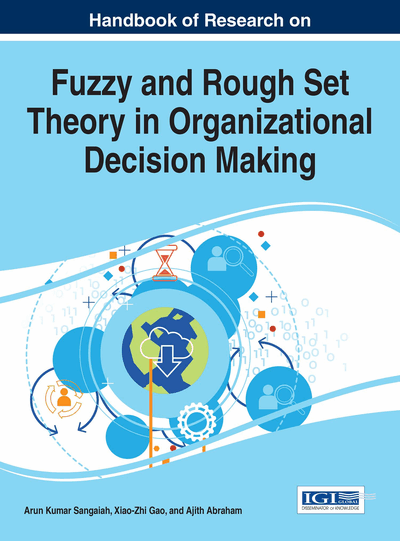# Parameter Reduction in Soft Set Models and Application in Decision Making

B.K. Tripathy (VIT University, India), R.K. Mohanty (VIT University, India), Sooraj T.R. (VIT University, India) and Arun K.R. (VIT University, India)
DOI: 10.4018/978-1-5225-1008-6.ch015

## Abstract

Soft sets were introduced by Molodtsov to handle uncertainty based problems. In 2003, Maji et al. extended the soft set approach to decision making. Decision making plays an integral role in daily life. In this chapter we describe soft sets and its impact in decision making problems. In this chapter, we discuss some of the basic notions of fuzzy soft sets and interval valued fuzzy soft sets. We also discuss application of fuzzy soft sets and interval valued fuzzy soft sets in decision making. In this chapter, we are going to see how parameter reduction is used in soft set theory.
Chapter Preview
Top

## Introduction

Soft set theory is a new mathematical approach to handle uncertainty based problems. It was introduced by the Russian mathematician D.A. Molodtsov (1999). Soft set theory is advanced by a number of researchers. Maji et al. (2001) has given the definition of fuzzy soft set and its application in decision-making problems (Maji et al., 2002). There are a lot of theories which deal with vagueness and ambiguity. Some important theories among those are probability theory, theory of interval mathematics and fuzzy set theory. All of these models are very good to handle uncertainty and vagueness in data. But each of these theories has their own drawbacks; such as fuzzy sets (Zadeh, 1965) are completely dependent on membership functions but there is no unique formula to define the membership functions. Molodtsov observed that the main reason of these drawbacks is perhaps due to the inadequacy of parameterization tools. That has led him to introduce soft set theory, which is a parameterized collection of subsets.

Most of the problems in real life contain uncertainties.When we handle real life situations, we can observe that most of the information available is ambiguous or uncertain. In real life, before we take any decision we have to collect sufficient information regarding with the problem. As the level of uncertainty increases in the problem, the decision making will become more complicated. In many cases, information is determined according to the decision maker’s. Decision maker should be an expert related with the problem. If the decision maker is not an expert in the corresponding field, then the decision he takes may contain lot of ambiguity. There are mainly three ways in which decision makers express their opinions. They are:

• 1.

Preference orderings

• 2.

Utility values.

• 3.

Preference relations.

Preference orderings are a collection of natural numbers which are permutation used by the experts showing the order positions of a set of alternatives in sequence. Utility values are a series of exact real numbers taken from a closed unit interval [0,1] to indicate the preferences of a decision maker towards different outcomes. Preference relation is constructed via pair-wise comparisons over the alternatives, and each value in it indicates the preference degree of one alternative over another.Out of the above mentioned methods, preference relation is much better. In the case of preference orderings, experts are not having sufficient information about the problem. In some cases, utility values are difficult to calculate. Preference relation is not having the above mentioned difficulties.

Broadly, we can classify decision making process into two categories, single decision making and group decision making process. In single decision making process, the decision will be taken by a single expert or decision maker. But, in most of the real life problems a single decision maker may not be enough to take a proper decision. So, the need of group decision making was introduced. In group decision making process, more than one decision maker evaluate the problem and take appropriate decision. But the decision makers need to have enough knowledge about the problem to take suitable decision. In Saaty (1986), Saaty introduced analytic hierarchy process. Analytic hierarchy process (AHP) is a structured technique for analyzing and organizing complex decisions. It has particular application in group decision making. It can be used in fields such as government, business, healthcare etc. The main aim of AHP is to find a solution which best suits their goals rather than the correct decision. In AHP, a complex multi criteria problem can be decomposed into a multi level hierarchic structure of objectives, alternatives etc. Then a scale is used to express the judgments in the form of pair-wise comparisons. The scale expresses the importance of the elements in a level with respect to the elements in the level immediately above it.

## Complete Chapter List

Search this Book:
Reset